# Face Recognition Door Lock System using Raspberry Pi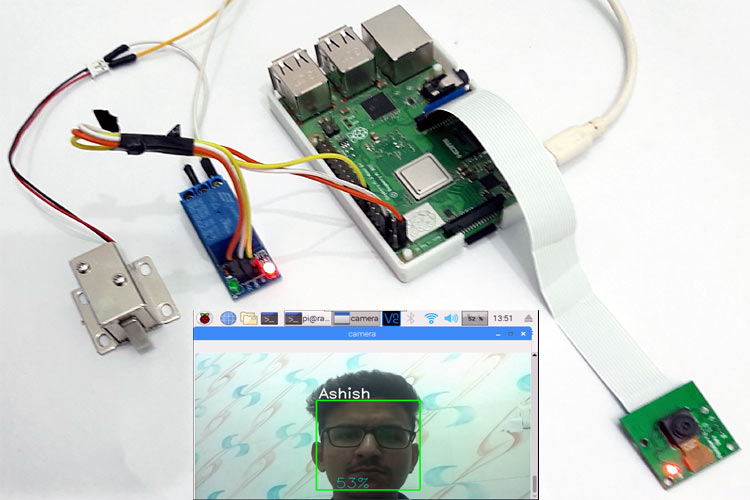Face Recognition technology has improved drastically in the past decade and now it is primarily used for surveillance and security purpose. In today’s tutorial, we will learn how to build the Face Recognition Door Lock System using Raspberry Pi. This project consists of three phases:

• Data Gathering
• Training the Recognizer
• Face Recognition

In the first phase, we will collect the face samples that are authorized to open the lock. In the second phase, we will train the Recognizer for these face samples, and in the last phase, trainer data will be used to recognize the faces. If raspberry pi recognizes a face, it will open the door lock.

Here, a solenoid lock and a Pi camera will be used with Raspberry Pi to build this face recognition-based door lock system using Raspberry Pi 3. We previously used solenoid lock with Raspberry pi, and also built few projects with Pi camera like Web Controlled Raspberry Pi Surveillance Robot, IoT based Smart Wi-Fi doorbell, Smart CCTV Surveillance System, etc.

### Components Required

• Raspberry Pi 3 (any version)
• Solenoid Lock
• Relay Module
• Jumper Wires

### Circuit Diagram

Circuit diagram for Face Recognition Door Lock using Raspberry Pi is given below.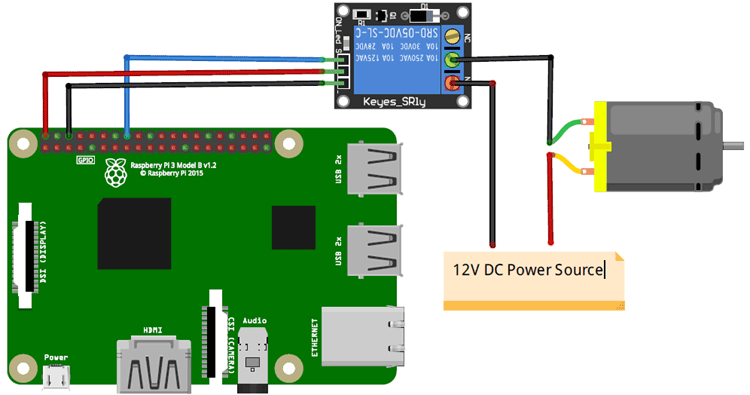Raspberry Pi and Solenoid Lock are connected through the relay module. Solenoid lock requires 9 to 12V, and Raspberry pi can provide only 5V. Due to this, a 12V adapter is used to power the Solenoid Lock. VCC and GND pin of the relay module is connected to 5V and GND of Raspberry Pi. The input pin of the relay is connected to GPIO23 of Raspberry Pi.

The positive pin of Solenoid lock is connected to the positive rail of the 12V adapter, while the negative pin of Solenoid Lock is connected to COM of Relay. Connect the NO pin of the relay to Negative of 12V adapter.

The breadboard setup for Raspberry Pi 3 Face Recognition Door Lock is shown below: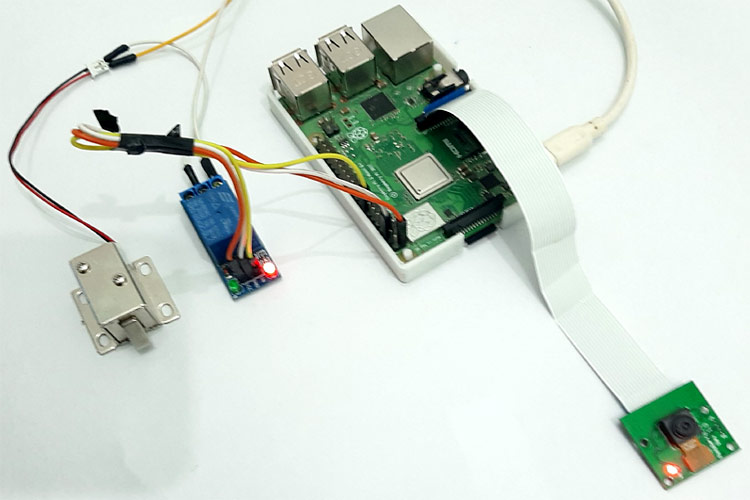### Installing OpenCV in Raspberry Pi 3

Here OpenCV library is used to detect and recognize faces. To install the OpenCV, first, update the Raspberry Pi.

`sudo apt-get update`

Then use the following commands to install the required dependencies for installing OpenCV on your Raspberry Pi.

```sudo apt-get install libhdf5-dev -y
sudo apt-get install libhdf5-serial-dev –y
sudo apt-get install libatlas-base-dev –y
sudo apt-get install libjasper-dev -y
sudo apt-get install libqtgui4 –y
sudo apt-get install libqt4-test –y```

After that, use the below command to install the OpenCV on your Raspberry Pi.

`pip3 install opencv-contrib-python==4.1.0.25`

### Installing other Required Packages for Face Recognition

Before beginning the program for Face Recognition Door Lock System using Raspberry Pi, let’s install the required packages.

Installing dlib: dlib is the modern toolkit that contains Machine Learning algorithms and tools for real-world problems. Use the below command to install the dlib.

`pip3 install dlib`

Installing face_recognition module: This library used to recognize and manipulate faces from Python through the command line. Use the below command to install the face recognition library.

`Pip3 install face_recognition`

Installing imutils: imutils is used to make essential image processing functions such as translation, rotation, resizing, skeletonization, and displaying Matplotlib images easier with OpenCV. Use the below command to install the imutils:

`pip3 install imutils`

Installing pillow: Pillow is used to open, manipulate, and save images in a different format. Use the below command to install pillow:

`pip3 install pillow`

### Programming for Raspberry Pi Face Recognition Door Lock

As mentioned earlier, we are going to complete this project in three-phase. The first phase is data gathering; the second is training the Recognizer, and the third is recognizing the faces. Programs for all three sections are given at the end. Here we have explained each one of them in detail.

1. Data Gathering

In the first phase of the project, create a Dataset to store the faces. These faces will be stored with different IDs.

For that, first, create a project directory where all the project data will be saved.

`mkdir FaceRecognition`

Besides the three python program and Dataset, this directory also has a Facial Classifier file. All three python programs and Facial Classifier file is given at the end of the page.

Now inside the FaceRecognitionProject directory, create a new subdirectory names Dataset to store the face samples.

`mkdir Dataset `

Now open a Nano editor file in FaceRecognitionProject directory and paste the data gathering program given at the end.

`sudo nano dataset.py`

Data Gathering program is explained below:

Initialize the face detector. The facial classifier file is used with a face detector.

`face_detector = cv2.CascadeClassifier('haarcascade_frontalface_default.xml')`

Now provide a user input command so that the user can enter the numeric face id before gathering the data.

`face_id = input('\n enter user id end press ENTER ==>  ')`

Inside the while loop, use the detector to extract the faces.

```ret, img = cam.read()
img = cv2.flip(img, -1) # flip video image vertically
gray = cv2.cvtColor(img, cv2.COLOR_BGR2GRAY)
faces = face_detector.detectMultiScale(gray, 1.3, 5)```

After that, save the each one of the captured frames, save it as a file on a "dataset" directory with the person id:

`cv2.imwrite("dataset/User." + str(face_id) + '.' + str(count) + ".jpg", gray[y:y+h,x:x+w])`

Run the python scripts and enter the face id. When it detects a face, it starts capturing the samples. These samples will be saved inside the Dataset directory.

This is how the directories and program files will be structured: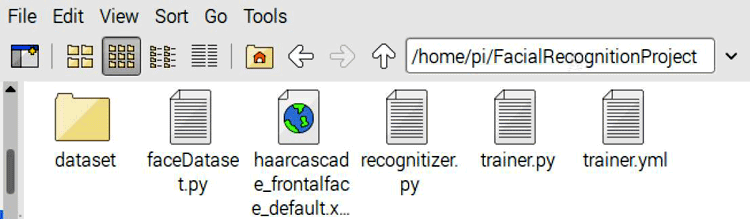2. Training the Recognizer

After gathering the face samples, train the Recognizer for these samples so that it can predict the faces accurately.

Open a Nano editor file inside the FaceRecognition directory, paste the trainer code (given at the end) and save it as trainer.py

Python script for training the Recognizer is explained below:

Start the code by importing all the required library files.

```import cv2
import numpy as np
from PIL import Image
import os```

After that, enter the path where you saved the face samples.

`path = 'dataset'`

Next, use the haarcascade_frontalface_default.xml facial classifier file to detect the faces in sample images. Then use the recognizer variable to create an LBPH (Local Binary Pattern Histogram) Face Recognizer.

```detector = cv2.CascadeClassifier("haarcascade_frontalface_default.xml");
recognizer = cv2.face.LBPHFaceRecognizer_create()```

Now get into the face samples directory using the path that is initialized earlier.

`imagePaths = [os.path.join(path,f) for f in os.listdir(path)]    `

After that, create two lists for storing face samples and IDs.

```faceSamples=[]
ids = []```

Convert the image samples into grayscale. After that, convert the PIL image into a numpy image.

```PIL_img = Image.open(imagePath).convert('L') # convert it to grayscale
img_numpy = np.array(PIL_img,'uint8')```

Sample in the Dataset directory is saved like this: User.Id.SampleNumber. So to get the ID, we will split the image path. By splitting the image path, we will get a User ID, and sample number.

`id = int(os.path.split(imagePath)[-1].split("."))`

Now call the Faces and IDs list and feed them into trainer file.

```faces,ids = getImagesAndLabels(path)
recognizer.train(faces, np.array(ids))```

3. Recognizer

Now in the final step of our project, we will use face recognition technology to recognize faces from the live video feed. Once raspberry pi recognizes any saved face, it will make the relay module high to open the solenoid lock.

The complete face recognition program is given at the end of the page. Some important parts of this code are explained below:

This program is similar to the trainer program, so use the same library files and also the classifier file.

After that, use an array to add the name for each face id.

`names = ['None', 'Ashish', 'Thor']`

Now obtain video feed from the raspberry pi camera in 640x480 resolution. If you are using more than one camera, then replace zero with one in cam = cv2.VideoCapture(0) function

```cam = cv2.VideoCapture(0)
cam.set(3, 640) # set video widht
cam.set(4, 480) # set video height```

After that, inside the while loop, break the video into images and then convert it to grayscale.

```ret, img =cam.read()
img = cv2.flip(img, -1) # Flip vertically
gray = cv2.cvtColor(img,cv2.COLOR_BGR2GRAY)```

Use recognizer.predict function to check how much the face matches with the samples.

```cv2.rectangle(img, (x,y), (x+w,y+h), (0,255,0), 2)
id, confidence = recognizer.predict(gray[y:y+h,x:x+w])```

If confidence is less than 100, then open the lock. 0 means the perfect match.

```if (confidence < 100):
id = names[id]
confidence = "  {0}%".format(round(100 - confidence))
GPIO.output (relay, 1)```

### Testing the Raspberry Pi Face Recognition Door Lock

Either an external monitor or any virtual monitor like VNC viewer will be needed to execute these python codes. Run the dataset program to gather the face samples. When you run this program, a window will pop-up. In that window, enter the ID number and press Enter. After that, another window will pop-up to take the face samples using the pi camera.

After this run the trainer program. Upon successful execution, it will generate a trainer.yml file into your project directory. This file will be used by Recognizer to recognize the face.

Now in the last step, run the recognizer program. If a face is recognized in the video feed, you will find a box around it with the name of the person like shown below: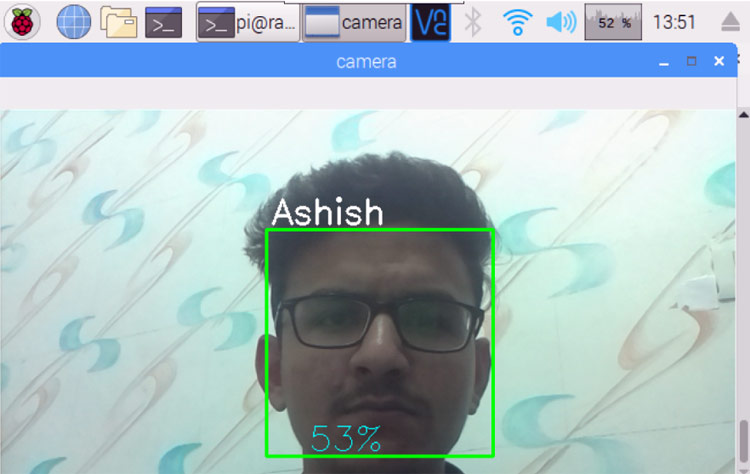A working video with all the python codes can be found at the end of the page.

Code

import cv2
import os
cam = cv2.VideoCapture(0)
cam.set(3, 640) # set video width
cam.set(4, 480) # set video height
# For each person, enter one numeric face id
face_id = input('\n enter user id end press <return> ==>  ')
print("\n [INFO] Initializing face capture. Look the camera and wait ...")
# Initialize individual sampling face count
count = 0
while(True):
img = cv2.flip(img, -1) # flip video image vertically
gray = cv2.cvtColor(img, cv2.COLOR_BGR2GRAY)
faces = face_detector.detectMultiScale(gray, 1.3, 5)
for (x,y,w,h) in faces:
cv2.rectangle(img, (x,y), (x+w,y+h), (255,0,0), 2)
count += 1
# Save the captured image into the datasets folder
cv2.imwrite("dataset/User." + str(face_id) + '.' + str(count) + ".jpg", gray[y:y+h,x:x+w])
cv2.imshow('image', img)
k = cv2.waitKey(100) & 0xff # Press 'ESC' for exiting video
if k == 27:
break
elif count >= 30: # Take 30 face sample and stop video
break
# Do a bit of cleanup
print("\n [INFO] Exiting Program and cleanup stuff")
cam.release()
cv2.destroyAllWindows()

import cv2
import numpy as np
from PIL import Image
import os
# Path for face image database
path = 'dataset'
recognizer = cv2.face.LBPHFaceRecognizer_create()
# function to get the images and label data
def getImagesAndLabels(path):
imagePaths = [os.path.join(path,f) for f in os.listdir(path)]
faceSamples=[]
ids = []
for imagePath in imagePaths:
PIL_img = Image.open(imagePath).convert('L') # convert it to grayscale
img_numpy = np.array(PIL_img,'uint8')
id = int(os.path.split(imagePath)[-1].split("."))
faces = detector.detectMultiScale(img_numpy)
for (x,y,w,h) in faces:
faceSamples.append(img_numpy[y:y+h,x:x+w])
ids.append(id)
return faceSamples,ids
print ("\n [INFO] Training faces. It will take a few seconds. Wait ...")
faces,ids = getImagesAndLabels(path)
recognizer.train(faces, np.array(ids))
# Save the model into trainer/trainer.yml
recognizer.write('trainer.yml') # recognizer.save() worked on Mac, but not on Pi
# Print the numer of faces trained and end program
print("\n [INFO] {0} faces trained. Exiting Program".format(len(np.unique(ids))))

import cv2
import numpy as np
import os
import RPi.GPIO as GPIO
import time
relay = 23
GPIO.setwarnings(False)
GPIO.setmode(GPIO.BCM)
GPIO.setup(relay, GPIO.OUT)
GPIO.output(relay ,1)
recognizer = cv2.face.LBPHFaceRecognizer_create()
font = cv2.FONT_HERSHEY_SIMPLEX
#iniciate id counter
id = 0
# names related to ids: example ==> Marcelo: id=1,  etc
names = ['None', 'Ashish', 'Loki']
# Initialize and start realtime video capture
cam = cv2.VideoCapture(0)
cam.set(3, 640) # set video widht
cam.set(4, 480) # set video height
# Define min window size to be recognized as a face
minW = 0.1*cam.get(3)
minH = 0.1*cam.get(4)
while True:
img = cv2.flip(img, -1) # Flip vertically
gray = cv2.cvtColor(img,cv2.COLOR_BGR2GRAY)
gray,
scaleFactor = 1.2,
minNeighbors = 5,
minSize = (int(minW), int(minH)),
)
for(x,y,w,h) in faces:
cv2.rectangle(img, (x,y), (x+w,y+h), (0,255,0), 2)
id, confidence = recognizer.predict(gray[y:y+h,x:x+w])
# Check if confidence is less them 100 ==> "0" is perfect match
if (confidence < 100):
id = names[id]
confidence = "  {0}%".format(round(100 - confidence))
GPIO.output(relay, 0)
print("Opening Lock")
#            time.sleep(1)
#           GPIO.output(relay, 1)
else:
id = "unknown"
confidence = "  {0}%".format(round(100 - confidence))
GPIO.output(relay, 1)
cv2.putText(img, str(id), (x+5,y-5), font, 1, (255,255,255), 2)
cv2.putText(img, str(confidence), (x+5,y+h-5), font, 1, (255,255,0), 1)
cv2.imshow('camera',img)
k = cv2.waitKey(10) & 0xff # Press 'ESC' for exiting video
if k == 27:
break
# Do a bit of cleanup
print("\n [INFO] Exiting Program and cleanup stuff")
cam.release()
cv2.destroyAllWindows()

Video